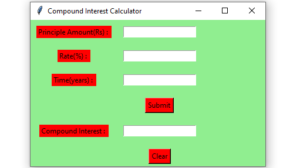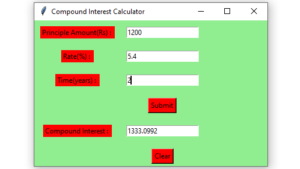# Python – Compound Interest GUI Calculator using Tkinter

Prerequisites : Introduction to tkinter | Program to calculate compound interest

Python offers multiple options for developing a GUI (Graphical User Interface). Out of all the GUI methods, Tkinter is the most commonly used method. In this article, we will learn how to create Compound Interest GUI Calculator application using Tkinter, with a step-by-step guide.

To create a Tkinter:

• Importing the module – tkinter
• Create the main window (container)
• Add any number of widgets to the main window.
• Apply the event Trigger on the widgets.

Let’s create a GUI based Compound Interest Calculator application :

Below is the implementation :

 `# import all classes / functions from the tkinter ` `from` `tkinter ``import` `*` ` `  `# Function for clearing the   ` `# contents of all entry boxes    ` `def` `clear_all() : ` ` `  `    ``# whole content of entry boxes is deleted  ` `    ``principle_field.delete(``0``, END)    ` `    ``rate_field.delete(``0``, END)  ` `    ``time_field.delete(``0``, END) ` `    ``compound_field.delete(``0``, END) ` `   `  `    ``# set focus on the principle_field entry box   ` `    ``principle_field.focus_set()  ` ` `  ` `  `# Function to find compound interest   ` `def` `calculate_ci():  ` ` `  `    ``# get a content from entry box ` `    ``principle ``=` `int``(principle_field.get()) ` `     `  `    ``rate ``=` `float``(rate_field.get()) ` ` `  `    ``time ``=` `int``(time_field.get()) ` `     `  `    ``# Calculates compound interest   ` `    ``CI ``=` `principle ``*` `(``pow``((``1` `+` `rate ``/` `100``), time)) ` ` `  `    ``# insert method inserting the   ` `    ``# value in the text entry box. ` `    ``compound_field.insert(``10``, CI) ` ` `  `     `  ` `  `# Driver code  ` `if` `__name__ ``=``=` `"__main__"` `:  ` `   `  `    ``# Create a GUI window  ` `    ``root ``=` `Tk()  ` `   `  `    ``# Set the background colour of GUI window  ` `    ``root.configure(background ``=` `'light green'``)  ` `   `  `    ``# Set the configuration of GUI window  ` `    ``root.geometry(``"400x250"``)  ` `   `  `    ``# set the name of tkinter GUI window  ` `    ``root.title(``"Compound Interest Calculator"``)   ` `       `  `    ``# Create a Principle Amount : label  ` `    ``label1 ``=` `Label(root, text ``=` `"Principle Amount(Rs) : "``,  ` `                   ``fg ``=` `'black'``, bg ``=` `'red'``)  ` `   `  `    ``# Create a Rate : label  ` `    ``label2 ``=` `Label(root, text ``=` `"Rate(%) : "``,  ` `                   ``fg ``=` `'black'``, bg ``=` `'red'``)  ` `       `  `    ``# Create a Time : label  ` `    ``label3 ``=` `Label(root, text ``=` `"Time(years) : "``,  ` `                   ``fg ``=` `'black'``, bg ``=` `'red'``) ` ` `  `    ``# Create a Compound Interest : label  ` `    ``label4 ``=` `Label(root, text ``=` `"Compound Interest : "``,  ` `                   ``fg ``=` `'black'``, bg ``=` `'red'``)  ` ` `  `    ``# grid method is used for placing   ` `    ``# the widgets at respective positions   ` `    ``# in table like structure . ` ` `  `    ``# padx keyword argument used to set paading along x-axis . ` `    ``# pady keyword argument used to set paading along y-axis .  ` `    ``label1.grid(row ``=` `1``, column ``=` `0``, padx ``=` `10``, pady ``=` `10``)   ` `    ``label2.grid(row ``=` `2``, column ``=` `0``, padx ``=` `10``, pady ``=` `10``)   ` `    ``label3.grid(row ``=` `3``, column ``=` `0``, padx ``=` `10``, pady ``=` `10``) ` `    ``label4.grid(row ``=` `5``, column ``=` `0``, padx ``=` `10``, pady ``=` `10``) ` ` `  `    ``# Create a entry box   ` `    ``# for filling or typing the information. ` `    ``principle_field ``=` `Entry(root)   ` `    ``rate_field ``=` `Entry(root)   ` `    ``time_field ``=` `Entry(root) ` `    ``compound_field ``=` `Entry(root) ` ` `  `    ``# grid method is used for placing   ` `    ``# the widgets at respective positions   ` `    ``# in table like structure . ` `     `  `    ``# padx keyword argument used to set paading along x-axis . ` `    ``# pady keyword argument used to set paading along y-axis .  ` `    ``principle_field.grid(row ``=` `1``, column ``=` `1``, padx ``=` `10``, pady ``=` `10``)   ` `    ``rate_field.grid(row ``=` `2``, column ``=` `1``, padx ``=` `10``, pady ``=` `10``)   ` `    ``time_field.grid(row ``=` `3``, column ``=` `1``, padx ``=` `10``, pady ``=` `10``) ` `    ``compound_field.grid(row ``=` `5``, column ``=` `1``, padx ``=` `10``, pady ``=` `10``) ` ` `  `    ``# Create a Submit Button and attached   ` `    ``# to calculate_ci function   ` `    ``button1 ``=` `Button(root, text ``=` `"Submit"``, bg ``=` `"red"``,   ` `                     ``fg ``=` `"black"``, command ``=` `calculate_ci)  ` `   `  `    ``# Create a Clear Button and attached   ` `    ``# to clear_all function   ` `    ``button2 ``=` `Button(root, text ``=` `"Clear"``, bg ``=` `"red"``,   ` `                     ``fg ``=` `"black"``, command ``=` `clear_all) ` `   `  `    ``button1.grid(row ``=` `4``, column ``=` `1``, pady ``=` `10``)  ` `    ``button2.grid(row ``=` `6``, column ``=` `1``, pady ``=` `10``) ` ` `  `    ``# Start the GUI   ` `    ``root.mainloop() ` `    `

Output :My Personal Notes arrow_drop_upCheck out this Author's contributed articles.

If you like GeeksforGeeks and would like to contribute, you can also write an article using contribute.geeksforgeeks.org or mail your article to contribute@geeksforgeeks.org. See your article appearing on the GeeksforGeeks main page and help other Geeks.

Please Improve this article if you find anything incorrect by clicking on the "Improve Article" button below.

Article Tags :

Be the First to upvote.

Please write to us at contribute@geeksforgeeks.org to report any issue with the above content.